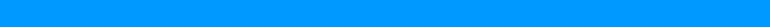# Computer Science 1033A/B Lecture Notes - Lecture 3: 5,6,7,8, English Alphabet, Octal

10 views7 pages
Page:
of 7COMP SCI – WEEK 3
Before computers, how did we encode data?
Before 1800s—drawings, paintings, books
after 1900s—photography, record albums
represent our thoughts—English words, pictures
represent our language—letters, words
how did we save representations of our thought before 1980ish—books, diaries, journals
How did we represent numbers in history?
How do humans rep. Cost and quantities—decimal #s
how did “the average person” calculate costs before home computers—calculators
how did we calculate costs before 1950s—slide ruler
how did we calculate costs before 1960s—abacus
why is our number system base 10? OR how did we calculate costs before 2700 BC—because
we used out fingers
How does computer represent number, words, images, and sound?
In a binary system
only uses 0 or 1
00 = 0
01 = 1
10 = 2
11 = 3
100 = 4
Computer only understand/speak binary
Representing characters in binary
use 1 byte for each letter
called ASCII code (assigns binary # for every letter)
with 8 bytes, or (1 byte), we can encode 2^8-->256 different characters
ASCII—only for English alphabet
Why do computers like binary?
A computer uses electricity and can distinguish getting a high volt or a low volt
high volt is a 1
low volt is a 0
Some basic concepts
everything we want to show/hear on a computer MUST be encoded
a lot of our world that was once measured in an analog manner can now be represented in a
digital manner
How many numbering systems are there?
Infinite!
Binary—(2 binary digits/BITS) 0, 1, 10, 11, 100, 101, 111, 1000
Octal—(8 digits) 0, 1, 2, 3, 4, 5, 6, 7, 10, 11, 12, 13, 14, 15, 16, 17, 20
Decimal—(10 digits) 0, 1, 2, 3, 4, 5, 6, 7, 8, 9, 10, 11, 12, 13, 14, 15, 16, 17, 18, 19, 20
Hexadecimal—(16 digits) 0, 1, 2, 3, 4,5 , 6, 7, 8, 9, A, B, C, D, E, F, 10, 11, 12, 13, 14, 15, 16,
17, 18, 19, 1A, 1B, 1C, 1D, 1E, 1F, 20...FA, FB, FC, FD, FE, FF, 100, 101, 102...FFE, FFF,
1000, 1001, 1002
as soon as you run out of patterns you need an extra place holder
Bit
means binary digit
8 bits = 1 byte
0 = 0
1 =1
10 = 2
11 = 3
100 = 4
101 = 5
110 = 6
111 = 7
1000 = 8
1001 = 9
1010 = 10
1011 = 11
Every time we need to increase the number of bits we are doubling the decimal
How many items can we represent with binary bits (how many patterns can we make)
if we have 1 bit—2 (0, 1)
if we have 2 bits—2x2 (00, 01, 10, 11)
if we have 3 bits—2x2x2 (000, 100, 001, 101, 010, 110, 011, 111)
Converting analog to digital
conversion is a 2 step process
step 1: sampling—how often do I take a sample (measurement) to represent the “thing”
(how many parts will I break the thing up into)
step 2: quantizing—how many discrete values will I use to represent the parts of the
“thing”
where “thing” mean image, sound, video, animation, text
the more chunks you break up an image into, the clearer it becomes
Quantizing for images
1 bit to represent the colour of each square (only 2 colours)--black and white
4 bits to represent the colour of each square (16 colours)--2^4 = 16 different shades
24 bits to represent the colour of each square (16 million)--2^24 = 16 million colour shades—
24 bit = 3 bytes for each chunk
Images great for
information
explanation
entertainment
Get images to computer
scanning existing images
taking picture with digital camera
the more dpi the better quality, but you take up more space
Terminology: DPI
when scanning or getting an image from our digital camera, the scanner, or the camera can
determine how many samples to take (how finely to break down the images)
the more samples that are taken, the higher the resolution will be
samples on scanner/printers are measured as dpi
samples on a monitor are measures as pixels per inch (PPI)
a sample in an image is called pixel
Pixel
means picture element
an image is represented by a grid (array matrix) of squared picture elements called pixels-pica
pixel is the smallest image component and thus shows the smallest detail
arranged in columns and rows
each pixel is given a numerical value that represents the corresponding colour
when printing an image, you should print with dpi of at least 300
Quantizing
giving colour to a picture
Digital Cameras
megapixels—how many millions of pixels you can capture in a photograph on your digital
camera
a camera with more megapixels can print a larger image without the human eye detecting a loss
of quality
if you just want to print 4” x 6” images, you don't need much more than 3 megapixels
Printing images on paper
when printing an image, the image must be printed at a size that has a minimum of 300 dpi
if an image was 3000 pixels by 1500 pixels, for the print quality to be good enough to the
human eye, what size should is print at?
answer: 3000/300—10 inches by 1500/300—5 inches. DO NOT print any larger than
10”x5”
Review questions
how many pixels is an image that is 800 pixels by 800 pixels?
800x800 = 640,000 pixels